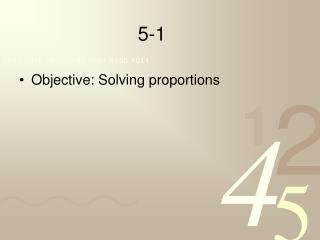DownloadDownload Presentation5-1

5-1

Télécharger la présentation5-1

- - - - - - - - - - - - - - - - - - - - - - - - - - - E N D - - - - - - - - - - - - - - - - - - - - - - - - - - -
Presentation Transcript

1. 5-1 • Objective: Solving proportions

2. An equation in which two ratios are equal is called a proportion. • A ratio is the comparison of two numbers written as a fraction. • A proportion can be written using colon notation like this a:b::c:d • Or as the more recognizable (and useable) equivalence of two fractions.

3. Proportions • To set up a proportion think part/whole part part ______ _____ = whole whole If you can travel 120 miles on 6 gallons of gas, how far can you drive on 8 gallons of gas? (8)gas (6)gas ______ ______ x=160 6x=960 = (x) miles 120miles

4. RATIOS & PROPORTIONS Are the following proportions? FALSE – Not a proportion FALSE – Not a proportion TRUE – this is a proportion TRUE – this is a proportion

5. RATIOS & PROPORTIONS Find the missing numbers to make the following proportions. 10 10 9 9

6. 5-2 • Objective: Solve proportions involving scale drawings and models

7. SCALE DRAWINGS • A scale drawing is a drawing of an object with dimensions proportional to those of the actual object. To find lengths from scale drawings, set up and solve proportions.

8. SCALE DRAWINGS USING PROPORTIONS EXAMPLE#1: ¼ in = 5 ft is a scale for a rock band’s concert stage. A. The scale length of the drum stand is 1 in. What is the actual length of the drum stand? Cross multiply. Divide by .25 .25 .25

9. SCALE DRAWINGS USING PROPORTIONS Given a scale of .5 in = 4 ft, find the actual length for each scale length given. .5 .5 .5 .5

10. 5-3 • Objective: Solve problems by using the percent equations

11. PERCENTS & DECIMALS • Percent (%) means per hundred. • To change a decimal to a percent • Multiply the decimal by 100 • Write a percent sign after it Multiply the decimal by 100(move the decimal point 2 places to the right) Write a percent sign after it

12. To change a percent to a decimal • Divide the percent by 100 • Omit the percent sign • Divide the percent by 100 (move decimal 2 places to the left) • Omit the percent sign

13. PERCENTS AND DECIMALS Convert the following decimals to percents: E. 0.445 44.5% A. 0.15 15% 1.5% F. 0.1111 11.11% B. 0.015 0.5% 62.5% G. 0.005 C. 0.625 104.2% H. 1.042 D. 0.9 90%

14. PERCENTS AND DECIMALS Convert the following percents to decimals: E. 1.5% 0.015 A. 23.45% 0.2345 0.25 F. 35% 0.35 B. 25% 0.00005 0.125 G. 0.005% C. 12.5% 3.1 H. 310% D. 45% 0.45

15. The percent equation P r = 100 B Percent r (percentage) = 100 Base

16. Figuring out what goes where? • Remember is/of • “is” will always be “P” • “of” will always be “B” What number is 15% of 10 What percent of 60 is 3 3 r P r = = 100 100 60 10